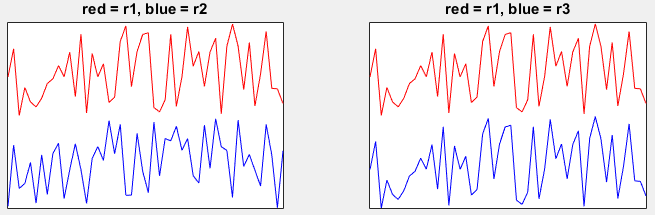Octave/Matlab  - Random Number                                    Home : www.sharetechnote.com

In this page, I would post a quick reference for Matlab and Octave. (Octave is a GNU program which is designed to provide a free tool that work like Matlab. I don't think it has 100% compatability between Octave and Matlab, but I noticed that most of basic commands are compatible. I would try to list those commands that can work both with Matlab and Octave). All the sample code listed here, I tried with Octave, not with Matlab.

There are huge number of functions which has not been explained here, but I would try to list those functions which are most commonly used in most of matlab sample script you can get. My purpose is to provide you the set of basic commands with examples so that you can at least read the most of sample script you can get from here and there (e.g, internet) without overwhelming you. If you get familiar with these minimal set of functionality, you would get some 'feeling' about the tool and then you would make sense out of the official document from Mathworks or GNU Octave which explains all the functions but not so many examples.

I haven't completed 'what I think is the minimum set' yet and I hope I can complete within a couple of weeks. Stay tuned !!!

Random Number Generation

< Single Random Number between 0 and 1 >

Case 1 : r = rand(1,1)

Ex)

 Input r = rand(1,1); Output r =          0.36579

< Single Random Number between a and b >

Case 1 : r = a + (b-a)*rand(1,1)

Ex)

 Input a = 2; b = 5; r = a + (b-a)*rand(1,1) Output r =          2.36579

< N Random Number between 0 and 1 >

Case 1 : r = rand(1,N)

Ex)

 Input r = rand(1,5); Output r =          0.199260   0.814680   0.687995   0.666070   0.013624

< N Random Number between a and b >

Case 1 : r = a + (b-a)*rand(1,N)

Ex)

 Input a = 2; b = 5; r = a + (b-a)*rand(1,5) Output r =          2.6419   4.1258   4.7051   2.0323   3.4711

< Single Random Integer between 0 and N >

Case 1 : r = randi(N)

Ex)

 Input r = randi(10) Output r =          5

< Single Random Integer between a and b >

Case 1 : r = randi([a b]) // where a and b are all integer

Ex)

 Input r = randi([100 200]) Output r =          163

< N Random Number between 0 and M >

Case 1 : ri = randi(M,1,N)

Ex)

 Input r = randi(100,1,10) Output r =           20   39   63   81   67   54   34   16   93   19

< N Random Number between a and b >

Case 1 : ri = randi([a b],1,N)

Ex)

 Input r = randi([100 200],1,10) Output r =           150   114   142   189   172   103   190   102   196   194

Case 1 : r = randn(1,N)

Ex)

 Input r = randn(1,5) Output r =            0.054629  -1.137793   0.736904  -0.326762   0.336358

Case 1 : r = randn(1,N) + m

Ex)

 Input r = randn(1,5) + 10 Output r =            9.4378    9.8131    9.6165   10.0008   10.4438

< N Random Number of Normal Distribution with mean =m, standard deviation = s >

Case 1 : r = sqrt(s)*randn(1,N) + m

Ex)

 Input r = sqrt(2)*randn(1,5) + 10 Output r =            10.1046    9.7616    7.7163   11.1219   10.2368

< N Binary Random Number with 0 and 1 >

Case 1 : r = randi([0 1],1,N)

Ex)

 Input r = randi([0 1],1,5) Output r =            1    0    0   1   1

< N Binary Random Number with -1 and 1 >

Case 1 : r = 2*randi([0 1],1,N)-1

Ex)

 Input r = 2*randi([0 1],1,5)-1 Output r =            1  -1  -1    1    1

< Complex Random Number with Re = {-1,1}, Im = {-1,1} >

Case 1 : r = (2*randi([0 1],1,N)-1)+j*(2*randi([0 1],1,N)-1)

Ex)

 Input r = (2*randi([0 1],1,5)-1)+j*(2*randi([0 1],1,5)-1) Output r =            -1 + 1i  -1 - 1i  -1 + 1i  -1 - 1i   1 - 1i

Case 1 : rng(SeedNumber)

Ex) In this example, r1 and r3 is generated with the same seed. So r1 and r3 should be same whereas r2 is different.

 Input N = 50; idx = 1:N;   rng(1); r1 = rand(1,N);   r2 = rand(1,N);   rng(1); r3 = rand(1,N);   subplot(1,2,1); plot(idx,r1 + 2,'r-',idx,r2 + 1,'b-'); xlim([1 N]); set(gca,'xtick',[]);set(gca,'ytick',[]); title('red = r1, blue = r2');   subplot(1,2,2); plot(idx,r1 + 2,'r-',idx,r3 + 1,'b-');xlim([1 N]); set(gca,'xtick',[]);set(gca,'ytick',[]); title('red = r1, blue = r3'); Output